﻿ Understanding some basic noise terms

# Understanding some basic noise terms

 Understanding some basic noise terms

There are terms you will come across over and over when working with fractals, noises and the like.

Understanding what these terms mean and their function inside a fractal or noise can help you understand any procedurals

behavior without much reading up in a user manual.

 Frequency

Frequency is a well established term and refers to the number of occurrences of a repeating event per unit.

In Computer Graphics, when generating a Noise it will start repeating itself at one point.

The Frequency determines the size/scale of the Noise features or in other words think of it like the "Texture Repeat" of the Noise.

Two different frequencies on the same noise. Notice the repeating noise pattern on the right.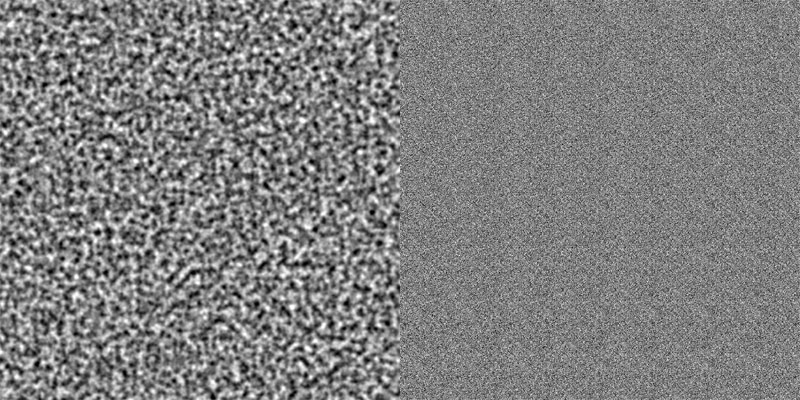Octaves

Octaves is probably the most important term to understand when working with any procedural.

It describes the number of loops that the code is run - making for finer and finer detail.

Most operations within a fractal or noise are run encapsulated within these loops.

Increasing Octaves on a simple noise results in more detail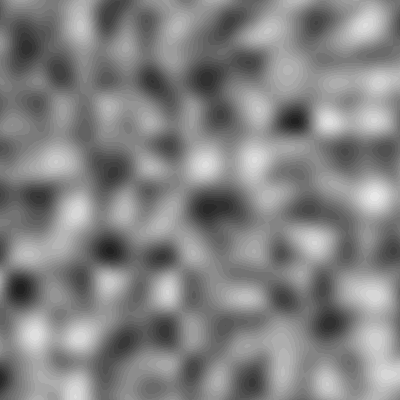Let's look at a simple example. In the below case we have 3 Octaves.

We feed in a Input value MyValue of 1.0 and we have set that within the loops MyValue should always have a value

of 0.1 added. The trick here is that MyValue will get larger and larger with each loop: For each loop add 0.1 to MyValue.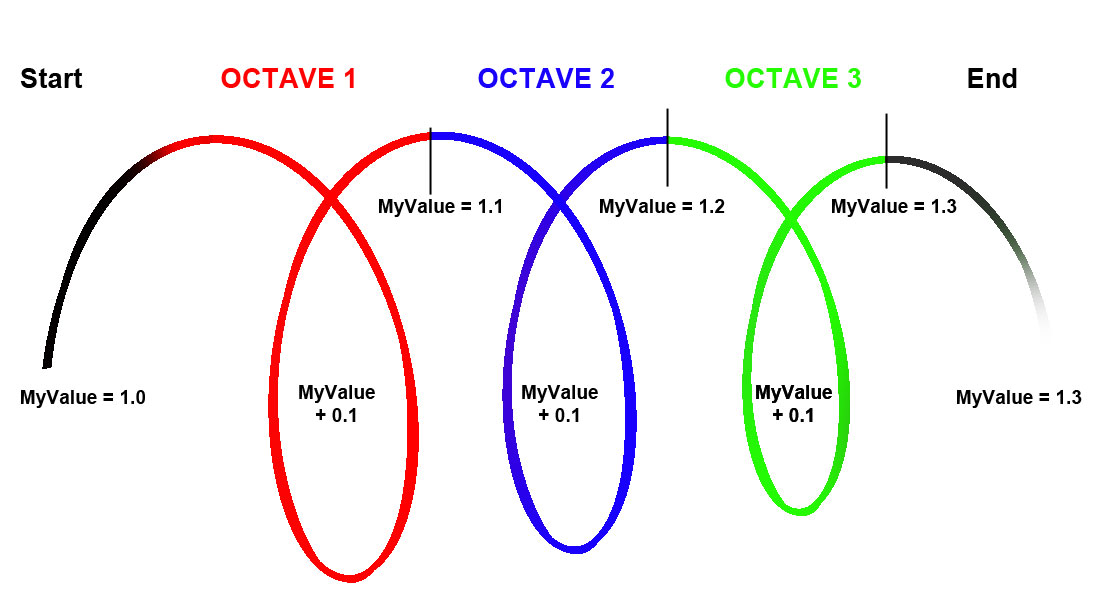Lacunarity

The term Lacunarity -  - refers to the 'texture' of a fractal or to be precise the general size of gaps (from the Latin lacuna meaning "gap")

In simple terms think of it like two noises with different sizes being overlayed making for finer and finer detail.

Changing the lacunarity on a simple noise.

Notice how it looks like a second changing fractal overlayed over the first.Amplitude

In the official wording Amplitude describes the maximum disturbance from the undisturbed position of a wave.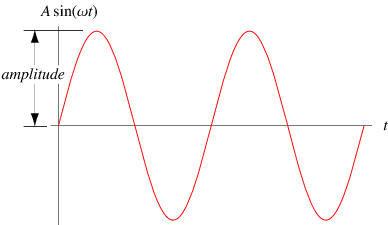In Computer Graphics Noises Amplitude often affects the length of the offset the Lacunarity is doing on the base pattern:

Increasing Amplitude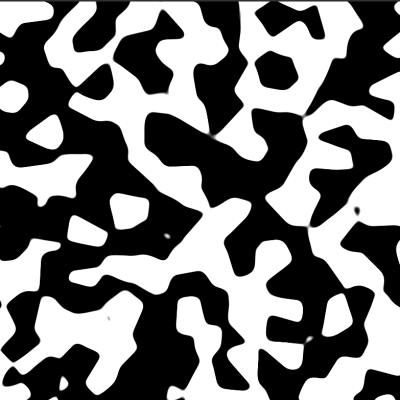Gain

Gain usually refers to a constant value being added to another one for each loop of an octave.

Where exactly the Gain can be different between noises but usually it is at least a multiplied value on the

Amplitude meaning that the Amplitude changes between octaves.

 Seed

Seed refers to a value that is used as a starting point to calculate the noise.

Changing the Seed usually changes the look of the noise without changing the look or size of its features.

 Fractalization

Fractalization refers to the process of nesting one Noise into another Noise. During each Octave of Noise A,

Noise B gets used to modulate Values of Noise A.

This leads to very complex and less repeating visual results since values get modified not only based on position in space

(the default noise way) but also change dramatically each loop of an octave.

 Multi Fractal

A multiFractal refers to a procedural that is generated out of different fractals.

You could wire one noise into attributes (such as the octaves, lacunarity etc.) of  another noise.

This adds complexity and reduces repetition on any procedural since instead of a constant value driving the specific attribute it is changing across your model / between pixels.

Created with the Personal Edition of HelpNDoc: Free PDF documentation generator